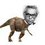# Projectile practice

You have a cannon a distance $d$ before the start of a hill. The hill goes up at an angle $\theta$ from the ground. What angle and velocity must you shoot a cannon ball so that it lands a distance $s$ up the hill?(The distance s is measured from the bottom of the hill, i.e. the distance along the hill).Note by Nihar Mahajan
6 years ago

This discussion board is a place to discuss our Daily Challenges and the math and science related to those challenges. Explanations are more than just a solution — they should explain the steps and thinking strategies that you used to obtain the solution. Comments should further the discussion of math and science.

When posting on Brilliant:

• Use the emojis to react to an explanation, whether you're congratulating a job well done , or just really confused .
• Ask specific questions about the challenge or the steps in somebody's explanation. Well-posed questions can add a lot to the discussion, but posting "I don't understand!" doesn't help anyone.
• Try to contribute something new to the discussion, whether it is an extension, generalization or other idea related to the challenge.

MarkdownAppears as
*italics* or _italics_ italics
**bold** or __bold__ bold
- bulleted- list
• bulleted
• list
1. numbered2. list
1. numbered
2. list
Note: you must add a full line of space before and after lists for them to show up correctly
paragraph 1paragraph 2

paragraph 1

paragraph 2

[example link](https://brilliant.org)example link
> This is a quote
This is a quote
    # I indented these lines
# 4 spaces, and now they show
# up as a code block.

print "hello world"
# I indented these lines
# 4 spaces, and now they show
# up as a code block.

print "hello world"
MathAppears as
Remember to wrap math in $$ ... $$ or $ ... $ to ensure proper formatting.
2 \times 3 $2 \times 3$
2^{34} $2^{34}$
a_{i-1} $a_{i-1}$
\frac{2}{3} $\frac{2}{3}$
\sqrt{2} $\sqrt{2}$
\sum_{i=1}^3 $\sum_{i=1}^3$
\sin \theta $\sin \theta$
\boxed{123} $\boxed{123}$

Sort by:

I think there are infinite solutions to this problem. There are infinite parabolas passing through (0,0) and $(d+sCos\theta,sSin\theta)$, the cannon at (0,0).

- 6 years ago

Well, I believe that the gravitational field is fixed, so you may assume that acceleration is $a$....

Staff - 6 years ago

Niranjan sir is right since angle and velocity both are upto us there are infinite possibilities.One of them has to be given for a finite(2 if velocity is given[not always]) number of solutions.

- 6 years ago

Oh yes that's true. For some reason, I though the angle of the cannon was fixed (when it's just the angle of the hill that is fixed).

So, what is the relationship between $\theta$ and $v$?

Staff - 6 years ago

I got $\large v= (d+s\cos\theta)\sqrt{\dfrac{g}{2(\sin 2\alpha)(d+s\cos\theta) - s\sin\theta\cos^2\alpha}}$ where $v$ and $\alpha$ is the required velocity and angle. As you say , there are infinitely many solutions. Is this correct?

This question is given to me by @Josh Silverman Sir.

- 6 years ago

The above equation has two unknowns. Only one equation. Thus infinity solutions.

- 6 years ago

Yes , that was what I was saying too.

- 6 years ago

Hey guys, I've made this into a well-constrained problem, here

Staff - 6 years ago